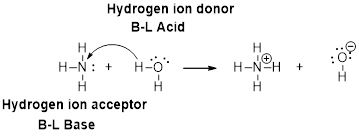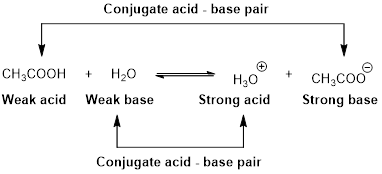Chapter 16, Problem 8PS

Chapter
Section
Textbook Problem

In each of the following acid-base reactions, identify the Brønsted acid and base on the left and their conjugate partners on the right. (a) C2H5N(aq) + CH3CO2H(aq) ⇄ C5H5NH+(aq) + CH3CO2−(aq) (b) N2H4(aq) + HSO4−(aq) ⇄ N2H5+(aq) + SO42−(aq) (c) [Al(H2O)6]3+ (aq) + OH−(aq) ⇄ [Al(H2O)5OH]2+ (aq) + H2O+(ℓ)

(a)

Interpretation Introduction

Interpretation:

Bronsted acid and base on the left and their conjugate partners on the right has to be identified.

C5H5N (aq) + CH3CO2H (l) C5H5NH+(aq) + CH3CO2-(aq)

Concept Introduction:

Bronsted –Lowry definitions:

A Bronsted –Lowry acid is a proton donor, it donates a hydrogen ion, H+

A Bronsted-Lowry base is a proton acceptor, it accepts a hydrogen ion H+.Bronsted –Lowry conjugate acid-Base pairs:

When an acid is dissolved in water, the acid (HA) donates a proton to water to form a new acid (conjugate acid) and a new base (conjugate base).Explanation

C5H5N (aq) + CH3CO2H (l) C5H5NH+(aq) + CH3CO2-(aq)

In the above reaction,

CH3COOH is donated a proton to C5H5N. Therefore, CH3COOH is a Bronsted acid.

C5H5N is accepted a proton from CH3COOH. Therefore,C5H5NH is a Bronsted base.

The conjugate base of CH3COOH is CH3COO- because it has one proton less than CH3COOH

(b)

Interpretation Introduction

Interpretation:

Bronsted acid and base on the left and their conjugate partners on the right has to be identified.

N2H4(aq) + HSO4- (aq) N2H5+ (aq) + SO42-(aq)

Concept Introduction:

Bronsted –Lowry definitions:

A Bronsted –Lowry acid is a proton donor, it donates a hydrogen ion, H+

A Bronsted-Lowry base is a proton acceptor, it accepts a hydrogen ion H+.Bronsted –Lowry conjugate acid-Base pairs:

When an acid is dissolved in water, the acid (HA) donates a proton to water to form a new acid (conjugate acid) and a new base (conjugate base).(c)

Interpretation Introduction

Interpretation:

Bronsted acid and base on the left and their conjugate partners on the right has to be identified.

[Al(H2O)6]3+(aq) + OH- (aq) [Al(H2O)5OH]2+(aq) + H2O (l)

Concept Introduction:

Bronsted –Lowry definitions:

A Bronsted –Lowry acid is a proton donor, it donates a hydrogen ion, H+

A Bronsted-Lowry base is a proton acceptor, it accepts a hydrogen ion H+.Bronsted –Lowry conjugate acid-Base pairs:

When an acid is dissolved in water, the acid (HA) donates a proton to water to form a new acid (conjugate acid) and a new base (conjugate base).Still sussing out bartleby?

Check out a sample textbook solution.

See a sample solution

The Solution to Your Study Problems

Bartleby provides explanations to thousands of textbook problems written by our experts, many with advanced degrees!

Get Started

Find more solutions based on key concepts